# Frame column stiffnessThe structural frame
The construction
The reinforcement I
The reinforcement II
Quantity/Cost estimation
Detailing drawings
Introduction >Wind and Seismic Forces >
Structural model and Analysis
Slabs
Seismic behavour of frames
Appendix A
Appendix B
Appendix C
Appendix D
Introduction >
Modelling slabsMaterials
To be continued >
Introduction

## Frame column stiffness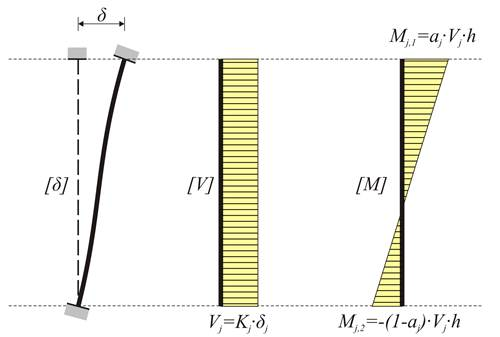Frame column stiffness in direction j (j=x, y):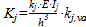Frames could comprise either columns only, called “frame systems” or columns and walls called “dual systems”. These two types of frame systems will be considered in the following paragraphs.

## One-storey frame structural systems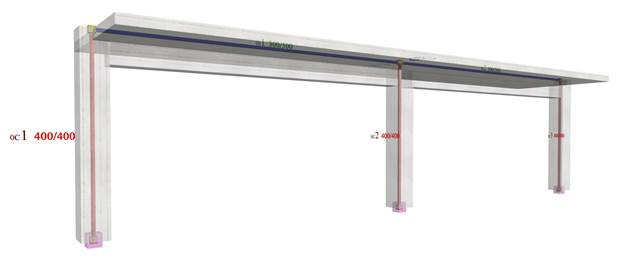The structural frame model without rigid bodies but fully stiffened

In project <B_5151a> of the related software, the cross-section of all columns is 400/400 and their height 3.0 m. The cross-section of the flanged beams is 300/500/1300/150 and their span 6.0 m. The seismic horizontal force is equal to 50 kN.

## Analysis results of project < B_5151a > with full beam and column stiffness (graphical representation in the related software)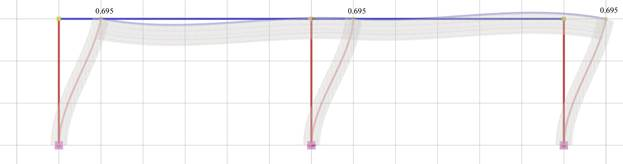Elastic line, δ=0.695 mm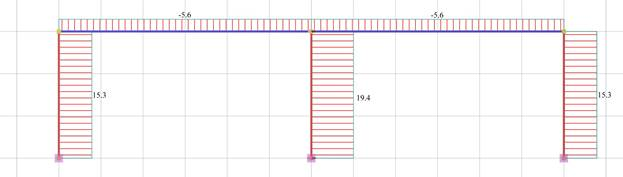Shear force diagramsBending moment diagrams

## Example of one-storey structural systems with foundationThe structural frame model fully stiffened, project <B_5151b>

Project <B_5151b> derives from project <B_5151a> by adding foundation beams with cross-section 300/800/1500/400 at level

-0.80 m

.

## Analysis results of project with actual beam and column stiffness (graphical representation in the related software)Elastic line, δ=1.480 mm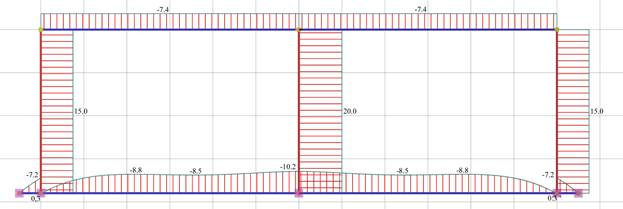Shear force diagrams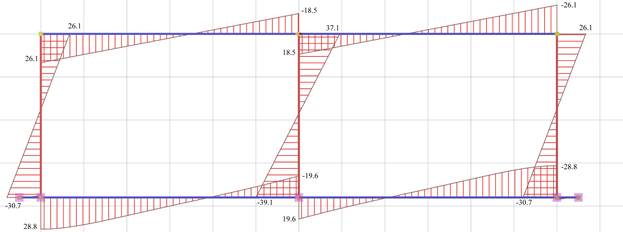Bending moment diagrams

## One-storey dual structural systemsOne-storey dual type structure comprising two columns and a wall (project <B_5152a>)

In project <B_5152a> of the related software, the structure comprises two end columns and a wall in the middle with cross-sections 400/400 and 2000/300 respectively. Their height is 3.0 m. The cross-section of the flanged beams is 300/500/1300/150 and their span is 6.0 m. The horizontal seismic force is50 kN.

## Analysis results of project <B_5152a> with actual beam and column stiffnesses (graphical representation in the related software)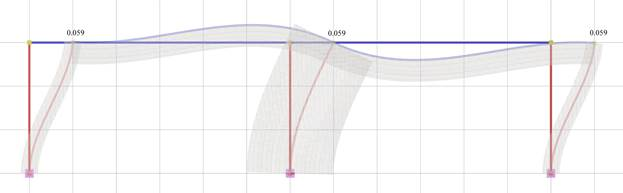Elastic line, δ=0.059 mm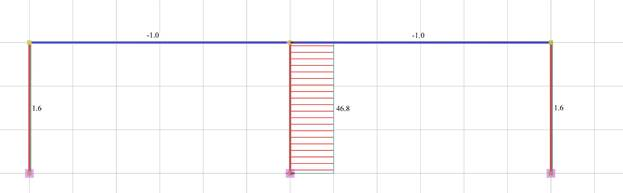Shear force diagramsBending moment diagram

## Example of a One-storey dual structural systems with foundationThe structural frame with the actual stiffness in project <B_5152b>

Project <B_5152b> derives from project <B_5152a> by adding at level -0.80 m flanged foundation beams with cross-section 300/800/1500/400.

## Analysis results of project with actual beam and column stiffness (graphical representation in the related software)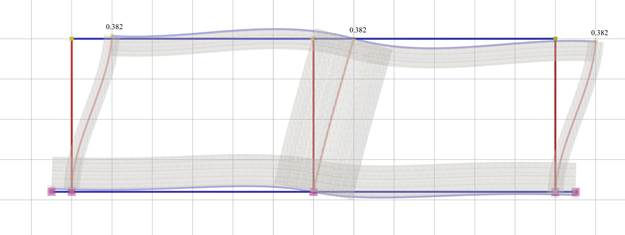Elastic line, δ=0.382 mmShear force diagramBending moment diagram